•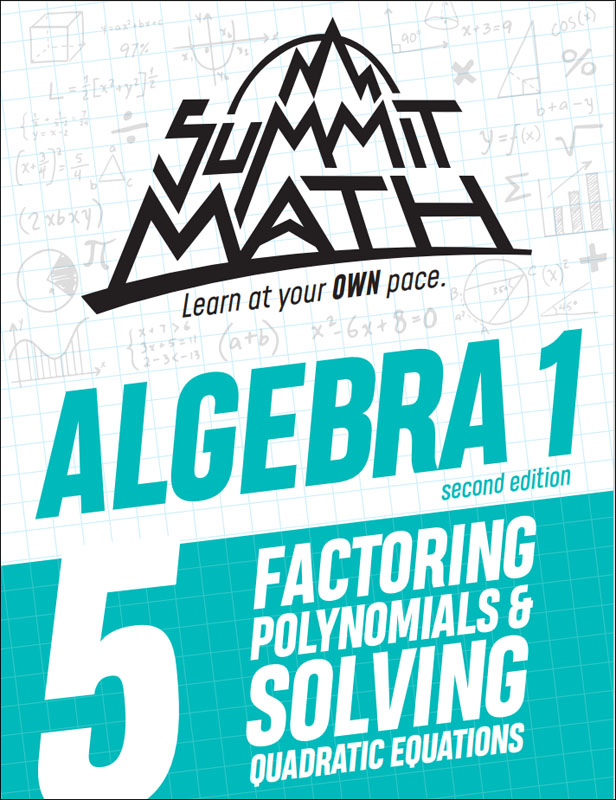•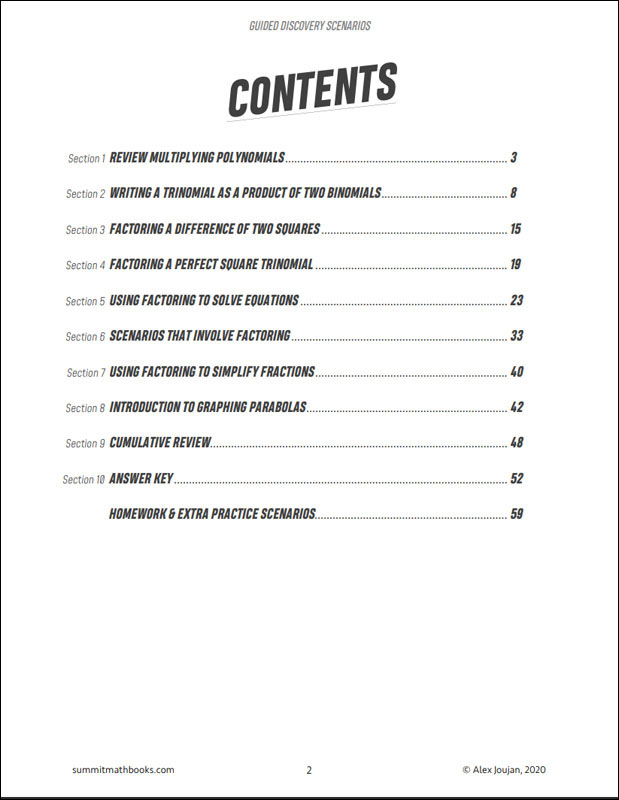•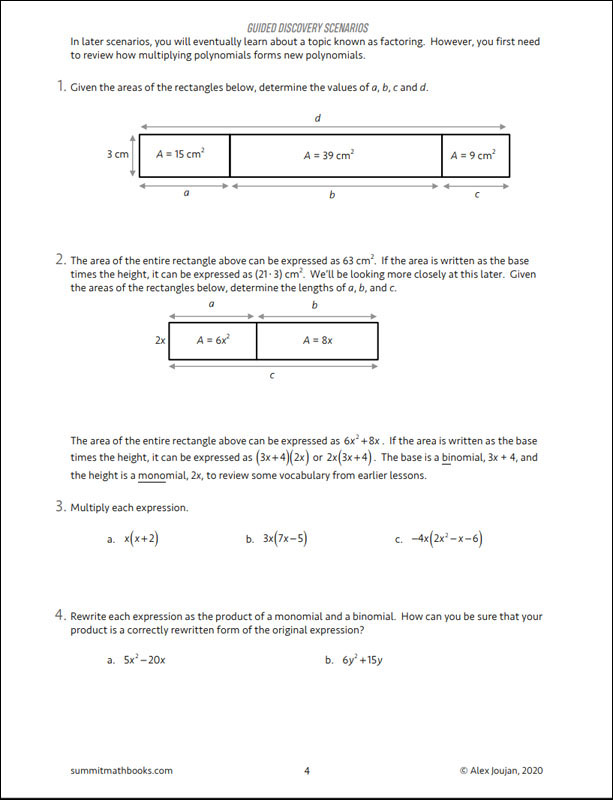•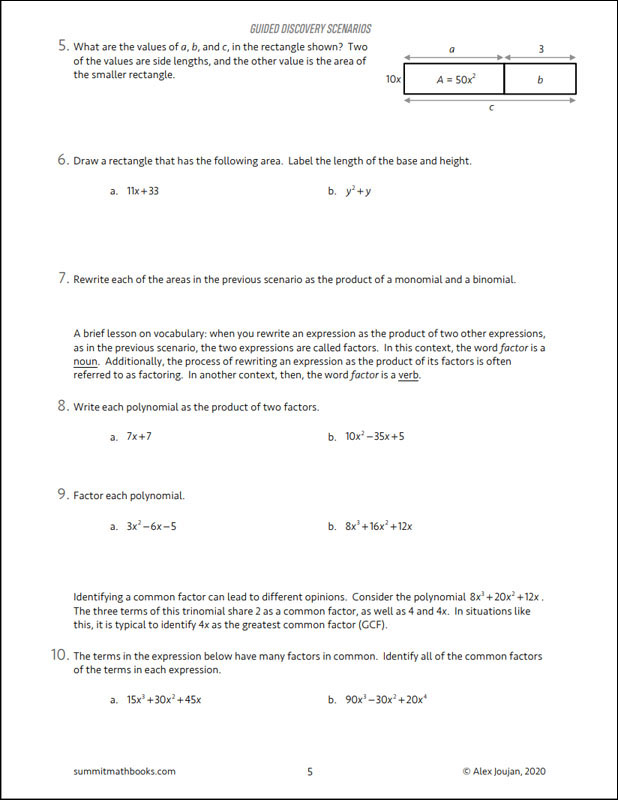•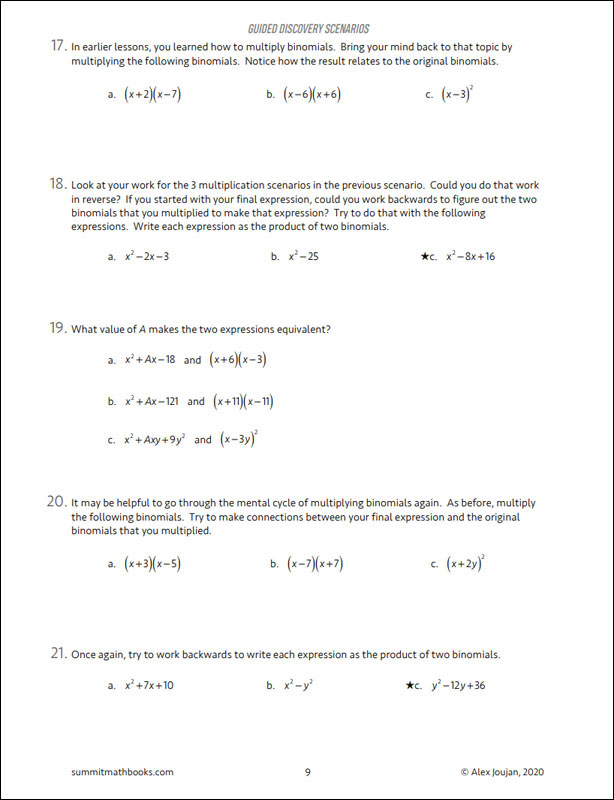# Summit Math Algebra 1 Book 5: Factoring Polynomials & Solving Quadratic Equations (2nd Edition)

# 078917

Our Price: \$12.25
Retail: \$12.50
Save: 2.00% (\$0.25)
More Stock expected soon. Available for Backorder.
Qty:
Qty:

Item #: 078917 9781712465745 6-10

#### Publisher Description:

This book builds on what students learn in Algebra 1: Book 4. Students learn how to think about multiplying polynomials in reverse order, which is known as factoring. They analyze factoring patterns that occur when a polynomial has a special structure like a difference of squares or a perfect square trinomial. They learn how to use factoring to solve quadratic equations and then they apply what they have learned as they solve a wide variety of scenarios that involve quadratic relationships. Near the end of the book, students are introduced to simplifying rational expressions, which they will study in more depth in Algebra 2: Book 4. They also learn about graphing parabolas, which they will study in more depth in Algebra 2: Book 3.

Topics in this book:

• Review multiplying polynomials
• Writing a trinomial as a product of two binomials
• Factoring a difference of two squares
• Factoring a perfect square trinomial
• Using factoring to solve equations
• Scenarios that involve factoring
• Using factoring to simplify fractions
• Introduction to graphing parabolas
• Cumulative Review

Category Description for Summit Math Algebra:

If you are searching for an independent algebra program or a supplement to teach specific algebra concepts, Summit Math is worth your consideration. Using the guided discovery method, students make their own connections as they learn to master each scenario before moving on to the next. Each section builds on previous concepts already learned. The first half of the book incorporates the guided discovery approach, while the latter half uses extra practice sheets to improve understanding of the topics. Each section has an answer key. A placement test and pacing guide is provided for each level. The author states this is a complete program teaching typical topics in the Algebra I and II sequence while preparing students for standardized testing. If students feel they need extra instruction, a video subscription is available from the publisher. Free downloadable tests and a more detailed version of the answer key are also available at the publisher’s website.

Primary Subject
Mathematics
6
10
ISBN
9781712465745
Format
Paperback
Brand Name
Summit Math
Weight
0.7 (lbs.)
Dimensions
11.0" x 8.5" x 0.25"